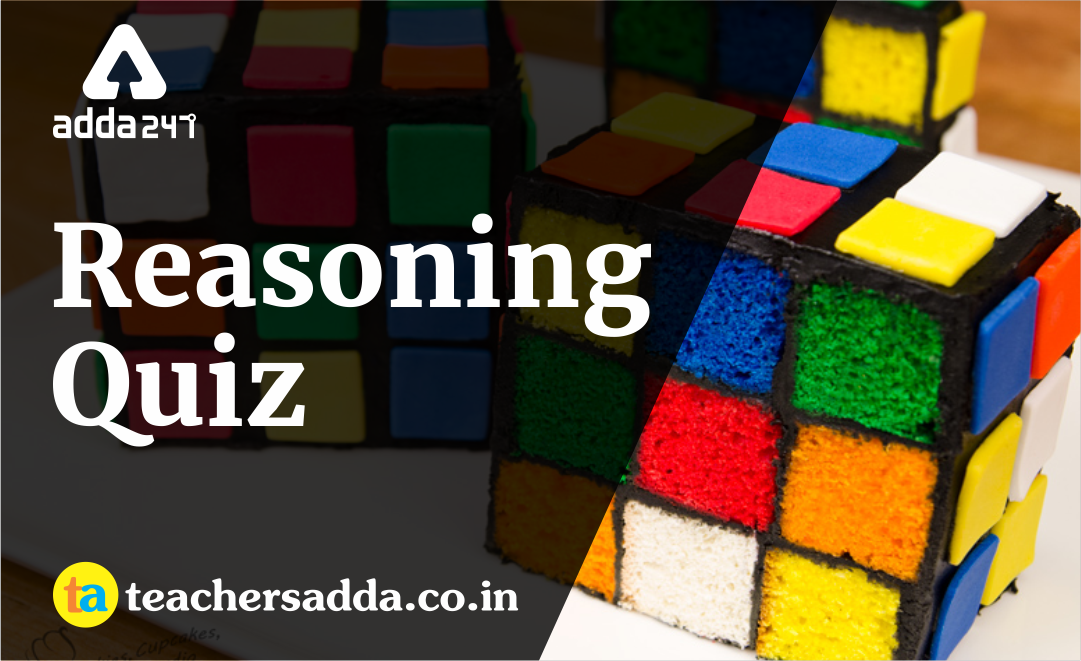The reasoning is the most scoring section in any competitive exam. It also develops or nourishes our critical thinking skills as compared to other subjects. In DSSSBKVSAPS Exam as a student to score well in this part, you have to start your preparation now because there is negative marking as well. The more you have prepared, the better you score.

Q1. If the ratio between the ages of P and Q is 2: 3 and that between the ages of Q and R is 4: 5, then the ratio between the ages of P and R is
(a) 3 : 4
(b) 3: 5
(c) 5: 6
(d) 8: 15

Q2. If W₁: W₂ = 2: 3 and W₁: W₃ = 1: 2, then W₂: W₃ is
(a) 3 : 4
(b) 4: 3
(c) 2: 3
(d) 4: 5

Q3. If one star equals four circles and three circles equal four diamonds, then what is the ratio of star to diamond?
(a) 1 : 3
(b) 3: 4
(c) 3: 16
(d) 16: 3

Q4. In a business, the ratio of the capitals of A and B is 2 : 1, that of B and C is 4 : 3 and that of D and C is 6 : 5. Then the ratio of the capitals of A and D is
(a) 9 : 20
(b) 3: 5
(c) 5: 3
(d) 20: 9

Q5. If A: B = 2: 3, B: C = 4: 5 and C: D = 6: 7, then A: B: C: D is
(a) 16 : 22 : 30 : 35
(b) 16 : 24 : 15 : 35
(c) 16 : 24 : 30 : 36
(d) 18 : 24 : 30 : 35

Q6. If x: y = 3: 1, then x³ – y³: x³ + y³ =?
(a) 10 : 11
(b) 11: 10
(c) 13: 14
(d) 14: 13

Q7. The fourth proportional to 5, 8, and 15 is
(a) 18
(b) 20
(c) 21
(d) 24

Q8. The third proportional to 38 and 15 isQ9. The product of the duplicate ratio of 2a: 6b and the reciprocal ratio of 4a²: 25b² is
(a) 0
(b) 1
(c) a: b
(d) None of these

Q10. Find the tow numbers whose mean proportional is 12 and the third proportional is 324.
(a) 6 and 8
(b) 4 and 36
(c) 3 and 24
(d) None of these

Solutions

S1. Ans.(d)
Sol.S2. Ans.(a)
Sol.S3. Ans.(d)
Sol.S4. Ans.(d)
Sol.S5. Ans.(c)
Sol.S6. Ans.(c)
Sol.S7. Ans.(d)
Sol.
Let the fourth proportional to 5, 8, 15 be x.S8. Ans.(a)
Sol.
Let the third proportional to 38 and 15 be x.S9. Ans.(d)
Sol.S10. Ans.(b)
Sol.Hence, the two numbers are 4 and 36.

You may also like to read :